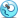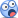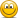Active Member
Hi,
A Futures Contract is said to be Trading Rich when :
the Actual Price E(St) > the Model Predicted Price = F0=S0* EXP[ ( Rf + Storage Costs ) - ( Div + Yield ) ] T--> Normal Contango

A Short Futures Contract would Deliver as Late as possible when (Div + Yield ) > ( Rf + Storage Costs) and this happens when Futures Contract Price is decreasing with time-> Backwardation

Would it be correct to tie the "Trading Rich" condition to the "Delivery as Late as possible"
and the "Trading Cheap" condition to the "Delivery as Early as possible"

David Harper CFA FRM

David Harper CFA FRM
Staff member
Subscriber
Hi @gargi.adhikari No, not exactly, but as usual, I am grateful to you for asking another smart question because it gives me a chance to improve the notes (in this case, by connecting different concepts). I just drafted the below (please give me any feedback because I do intend to add it to the Hull notes in Chapter 5 summary). The idea with this graphic is that the two dark circles (green and blue) represent currently observed (ie, traded) prices: S(0) and F(0,T). The lighter circles are theoretical and unobserved, they are model outputs not traded prices: theoretical F(0,T) and E[S(t)]. In this way, consider the following:
• The most basic forward curve phenomenon is its observed shape. If it is upward-sloping such that observed F(0, T++) > F(0,T) > S(0) we call this contango; if it is downward-sloping such that observed F(0, T++) < F(0,T) < S(0) we call this backwardation or simply "inverted."
• If observed F(o,T) < E[S(t)], we call this "normal backwardation" and, strictly (following Don Chance/CFA), F(o,T) > E[S(t)] we call this "normal contango." Note this is an unobserved (and arguably, un-provable) phenomenon. Also note, per the four light E[S(t)] that "normal backwardation | contango" is independent of contanto or backwardation. We can have four different permutations, although theory expects "normal backwardation" and "contango."
• The cost of carry model, in its universal form given by F(0,T) = S(0)*exp[(r+ u - q - y) produces for us the theoretical futures price which is displayed below as the light blue F(0,T), as opposed to the observed F(0,T). Notice that the theoretical price can be above or below the observed price, independent of whether the observed forward curve is in contango or backwardation!
• With respect to the the short position's choice to deliver, Hull argues that it's a function of the observed forward curve: if the observed forward curve is in contango, the short should deliver as soon as possible (and "futures prices in these circumstances should be calculated on the basis that delivery will take place at the beginning of the delivery period."). The rationale is that carrying (owning the asset) is expensive over time (i.e., c > y), so you'd want to get rid of it as soon as possible. On the other hand, if the observed curve is inverted (aka, in backwardation), Hull says the short wants to delay delivery as long as possible because the convenience of ownership apparently is outweighing the costs.
• With respect to "trading cheap" or "trading rich," these terms always refer to the relationship between a model (output) price and an observed price, so here "trading rich" is when the theoretical F(0,T) price is less than the observed F(0,T), as in (eg) "the observed December futures price is higher than my model says it should be under my model's assumptions." Notice that trading rich/cheap is independent of contango/backwardation.
I hope that's a helpful summary! I'll take any feedback because this is a draft of what I'll add to the next version of the Hull note. Thanks,Active Member
@David Harper CFA FRM Thanks so much again for patiently laying out the above ...Completely agree with all of the Bullet Points you laid out...The above helped clear our out some of the fuzziness I had on the above and the differences/nuances in each of the above scenarios.

Active Member
@David Harper CFA FRM On second thoughts- have a follow up question ...
So When ( Div + Yield ) > ( Rf + U ) = > F(0,T) < E(St) => Normal Backwardation
Also, When ( Div + Yield ) > ( Rf + U ) , the Short should Deliver as Late-As-Possible

So, isn't The Choice-of-Delivery is tied to Normal-Backwardation & Normal- Contango instead of being tied to just Contango or Backwardation...?

David Harper CFA FRM

David Harper CFA FRM
Staff member
Subscriber
Hi @gargi.adhikari I don't think that is exactly so, please see below. It is true that "normal backwardation" refers to F(0,T) < E[S(t)] but when (q+y) > (r +u), then the COC models prices F(0,T) < S(0) per F(0) = S(0)*exp[(r+u-q-y)*T] but that's just "backwardation"; please note that it is not necessarily true that F(0) = E[S(t)]. My learning XLS actually never has them equal because it assumes Hull's theory of normal backwardation such that, if the commodity beta β>0, then F(0) < E[S(t)]. The "theory of normal backwardation" says that F(0) = E[S(t)] only if the commodity has zero beta.

So if (q+y) > (r+u) then the theoretical futures price, F(0,T) < S(0). Further, we also expect the actual F(0,T) < S(0) although it can still "trade rich or cheap" which concerns the relationship between theoretical F(0) and trading F(0). Technical note: the convenience yield is subtle: if we are using the traded futures price to necessarily infer the convenience yield, then (q+y) > (r+u) must refer to the implied, observed backwardation (i.e., the dark circles). But neglecting this nuance, the inequality (q+y) > (r+u) is generally implying an observed backwardation (it does not imply a normal backwardation). So, when (q+y) > (r+u), we can expect a backwardation + normal backwardation, but we could still have a backwardation + normal contango!Last edited:

Active Member
Thanks @David Harper CFA FRM I guess am a bit fuzzy on some of the formulae which I need to clarify first... :-(

So, is F0 = E(St). EXP (r- k) T , k= being the growth rate = (Div + Yield)
Or is, F0 = S0. EXP (r- k).T ............... ?Where, S0 = The Spot-Price at Time =0 and
E(St)= The Expected-Future-Spot-Price at Time =t = S0. EXP (k.T ) , k= being the growth rate =or the Expected Rate of Return

Also, is S(t ) the same as E( St) both being = The Expected-Future-Spot-Price at Time =t = S0. EXP (k.T ) or are they different...?Last edited:

brian.field

Well-Known Member
Subscriber
Thanks @David Harper CFA FRM I guess am a bit fuzzy on some of the formulae which I need to clarify first... :-(

So, is F0 = E(St). EXP (r- k) T , k= being the growth rate = (Div + Yield)
Or is, F0 = S0. EXP (r- k).T ............... ?Where, S0 = The Spot-Price at Time =0 and
E(St)= The Expected-Future-Spot-Price at Time =t = S0. EXP (k.T ) , k= being the growth rate =or the Expected Rate of Return

View attachment 1301

The sub 0 indicates time 0. So F_o is the forward price now, e.g. at time 0, so we could more accurately call this the prepaid forward price. This prepaid forward price must equal the current stock price to avoid arbitrage. We know the stock price now so there is no "expectation". This price must also equal the present value of the forward price. If we take the prepaid forward price and accumulate forward to time t at risk free rate minus dividend yield, we get the forward price at time t.

brian.field

Well-Known Member
Subscriber
At time t, the coefficient is the expected value of S at time t, e.g. S_t

David Harper CFA FRM

David Harper CFA FRM
Staff member
Subscriber
@brian.field right, we cannot observe S(t) so there is really only an E[S(t)] and an observed S(0). But F(0) <> S(0) <> E[S(t)]. COC says F(0) <> S(0) and theory of normal backwardation says F(0) < E[S(t)] when the commodity has positive beta.

Active Member
Thanks @brian.field @David Harper CFA FRM so much for correctly pointing out the difference between F0 and F( 0, T) ...but my confusion is on the discrepancy I see in the formula for Fo.
So, is F0 = E(St). EXP (r- k) T , k= being the growth rate = (Div + Yield)
Or is, F0 = S0. EXP (r- k).T ............... ?Also, at time= t, the coefficient is the expected value of S at time t ....so that is E( St) ...right ..? is this the same as St...? or is E(St) different from St.... ?

David Harper CFA FRM

David Harper CFA FRM
Staff member
Subscriber
@gargi.adhikari I'm not aware of a difference between F(0) and F(0,T)?? ie., both are today's future price, but F(0,T) is better notation because it's giving us the time to maturity. There is an observed (aka, traded) forward price F(0,T) and a theoretical F(0,T) price impled by the COC model.

Last edited:

David Harper CFA FRM

David Harper CFA FRM
Staff member
Subscriber
@gargi.adhikari Under the theory of normal backwardation (ie, Hull's discussed approach), F(0) = E[S(t)]*exp[(r-k)T] where (k) is a the discount rate and is given by CAPM: k = r + β(commodity, M)*ERP; i.e, if β>0, then k > r, and F(0) < E[S(t)]. So, again, just to parrot Hull:
• the COC model tells us about the theoretical futures price F(0,T) as a function of the current spot price, S(0). As a first-order matter, it doesn't speak to E[S(t)] and doesn't utilize the discount rate (k)
• The theory of normal backwardation concerns the relationship between F(0,t) and E[S(t)] and utilizes the discount rate (k) because it posits that hedgers (who are net short) are paying speculators to transfer the systematic risk, β, to the speculators who are net long the futures contracts.

Last edited:

Active Member
@gargi.adhikari I'm not aware of a difference between F(0) and F(0,T)?? ie., both are today's future price, but F(0,T) is better notation because it's giving us the time to maturity. There is an observed (aka, traded) forward price F(0,T) and a theoretical F(0,T) price impled by the COC model.
@David Harper CFA FRM Thanks so much for reminding of the above...going back to this topic after a while and seeing the 2 different notations caused some some confusion on my part.. :-( Wish all the Finance Authors..Hull, Tuckman etc...followed some standard Notation/Terminology.... :-(

I had thought F0= to be the Futures Price Observed Today for Delivery at Time= 0= today
and F( 0, T) = to be the Futures Price Observed Today for Delivery at Time= t ...But I guess thats not the case...? F( 0, T) stands for F0....

So can you please confirm if I am understanding this correctly...
Case A : If F0 is to be expressed in terms of the Discount Rate, then we express F0 as a function of E(St) which is the Expected Future Spot Rate => F(0) = E[St]*exp[(r-k)T
Case B : Else, By the COC Model, Fo is a Function of the S0 which is the Current-Spot -Rate=> F0= S0* EXP[ ( Rf + Storage Costs ) - ( Div + Yield ) ] T

Again, my apologies for bugging you all so much on this topic and infinite gratitude for your patience with me on this topic...but am glad I asked to clarify or else I would have had a serious flaw in my understanding of the above....but still would wait to see if I finally got this right or I still am missing something...David Harper CFA FRM

David Harper CFA FRM
Staff member
Subscriber
Hi @gargi.adhikari It's no problem, it's true the notation between authors is not consistent, but it's also true we probably can't understand all of this without understanding the notation. Sometimes, when I get stuck on the math, I find the best thing I can do is slow down, take a step backwards and re-read the more fundamental setup to a concept. In this case, as far as I am concerned F(0) is just an abbreviated form of F(0,T); Hull tends to use the former, and McDonald the latter. But they are always synonyms, in my experience. It is currently August, if I look at the corn futures prices (see http://www.cmegroup.com/trading/agricultural/grain-and-oilseed/corn.html) I see that the December 2017 contract has a recent price of $3.64 per bushel. As December is four months forward, we can represent this observed (aka, traded) price with: F(0, 4/12) = F(0, 0.33) =$3.64; i.e., we can enter the contract to buy corn in December and pay $3.64 in December, or we can promise to deliver corn in December and receive$3.64 in December. Imagine now stepping forward in time one month (ie, imagine we travel forward to September): this same contract's price, this contract for December delivery, will be signified by F(0, 3/12) = F(0, 0.25). If you haven't yet, I strongly encourage looking at http://www.cmegroup.com/trading/agricultural/grain-and-oilseed/corn.html ... we can see there is currently a futures corn curve: F(0, 1/12) = $3.51; F(0, 4/12) = 3.64; F(0, 8/12) =$3.77. All of these are currently observed (traded prices) which is the meaning of the '0' in F(0,T).
• In Hull's cost of carry chapter and model, as the exploration is largely around a single future time interval, T, the use of F(0) is just an abbreviation of F(0,T).
• Re: "I had thought F0= to be the Futures Price Observed Today for Delivery at Time= 0= today:" this should be the spot price, S(0). As we approach maturity--ie., as (T) decreases in F(0,T)--we expect the futures price to converge to the spot price. But otherwise, we specifically do not expect F(0,T) to equal S(T). If (T) = 4 months or 0.33 years, then please notice, there is no currently observed such thing as S(0.33); i.e., we cannot currently observe the spot price of corn in December. But we can have an expected future spot price of corn in December, denoted E[S(t)] or E[S(T)]. But we only expect F(0) = E[S(t)] if r = k (ie., no systematic risk).
• Case A: F(0) = E[St]*exp[(r-k)T is correct and this is the perhaps more "theoretical" because it involves un-observable factors, namely E[S(t)] but also the discount factor (k) is not as observable as, say, the storage cost of corn.
• Case B: F0= S0* EXP[( Rf + Storage Costs ) - ( Div + Yield ) * T] is also correct as the COC model; I moved your "T" inside the square brackets.
So please note, from the beginning of this thread, I have maintained there are three key variables really:
• Theoretical F(0,T) which is given by the COC model
• Observed F(0,T) which is the traded price and, if it different than theoretical F(0,T), they we say it is "trading rich" or "trading cheap" (and this is a general idea: trading rich/cheap refers to an traded-observed price that doesn't match the model price, and is to be expected if for no other reason that the technical factors like supply/demand but also fundamental factors specifically, the model may be incomplete. Options can trade cheap/rich, bonds can trade cheap/rich. These are statements comparing a model output to an observed price).
• Expected future spot price, E[S(t] ... and, of course there is S(0)
If you like, here is a draft of my commodity COC model (that will be in the next version of the massive Hull learning XLS, it builds on the current version in the Hull XLS): https://www.dropbox.com/s/590uw43t29ey0ap/0817-commodity-lease.xlsx?dl=0
... but this is only for intermediate/advanced viewing please: there are still some little kinks to address

David Harper CFA FRM

David Harper CFA FRM
Staff member
Subscriber
Hi @gargi.adhikari Yes, you are completely correct. Sorry, I hesitated to share the sheet on the forum because, as I mentioned, it is a draft and "there are still some little kinks to address." It does not yet check for usability "errors" like the one you cite. Cell D20 is highlit yellow to indicate that it will be hard-coded (to reflect it could really be anything as a "traded" number). I had its value as "=D17" set only temporarily. C21 currently contains =IF(D20<D17,"Cheap","Expensive") but is not robust, and it should contain (something like) =IFS(D20=D17,"Fair",D20<D17,"Cheap",D20>D17,"Expensive"). In which case, to your point, if its value happens to exactly equal the theoretical F(0), then it will return "Fair." As you suggest, if the "Observed Forward Price" (cell 20) equals the Theoretical F(0), then it should return "fair" instead of "cheap" or "expensive". BTW, another small glitch in the current version is that, when the Observed Forward Price is set to equal the theoretical forward price, the cash and carry arbitrage does not produce a final net profit of exactly zero (instead it is -\$0.00041 under any future stock price). The near-zero profit is robust to any future stock price, which is cool and I am confident the basic operation is correct, but I think it should be calculating to exactly zero (I expect it has something to do with my conversion of storage and convenience into lumpy sums .... but maybe not ....). In any case, sorry the XLS gave you pause, however, to be honest, if you looked at the XLS and the XLS makes sense to you, then you probably understand this very wellgiven that you managed to find one of the few glitches in the XLS. Thank you!

Last edited:

@David Harper CFA FRM Thanks so much David for the clarification- Thanks to you I was able to clear my concepts on this topic